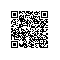# Java笔记3：Java精确计算

public class Test{
public static void main(String args[]){
System.out.println(0.05+0.01);
System.out.println(1.0-0.42);
System.out.println(4.015*100);
System.out.println(123.3/100);
}
};

0.060000000000000005
0.5800000000000001
401.49999999999994
1.2329999999999999
Java中的简单浮点数类型float和double不能够进行运算。不光是Java，在其它很多编程语言中也有这样的问题。在大多数情况下，计算的结果是准确的，但是多试几次（可以做一个循环）就可以试出类似上面的错误。现在终于理解为什么要有BCD码了。

public double round(double value){
return Math.round(value*100)/100.0;
}

4.015*100=401.49999999999994

java.text.DecimalFormat也不能解决这个问题：
System.out.println(new java.text.DecimalFormat("0.00").format(4.025));

BigDecimal

BigDecimal(double val)
Translates a double into a BigDecimal.
BigDecimal(String val)
Translates the String repre sentation of a BigDecimal into a BigDecimal.

Note: the results of this constructor can be somewhat unpredictable. One might assume that new BigDecimal(.1) is exactly equal to .1, but it is actually equal to .1000000000000000055511151231257827021181583404541015625. This is so because .1 cannot be represented exactly as a double (or, for that matter, as a binary fraction of any finite length). Thus, the long value that is being passed in to the constructor is not exactly equal to .1, appearances nonwithstanding.
The (String) constructor, on the other hand, is perfectly predictable: new BigDecimal(".1") is exactly equal to .1, as one would expect. Therefore, it is generally recommended that the (String) constructor be used in preference to this one.

public static double add(double v1,double v2)
public static double sub(double v1,double v2)
public static double mul(double v1,double v2)
public static double div(double v1,double v2)
public static double div(double v1,double v2,int scale)
public static double round(double v,int scale)

import java.math.BigDecimal;
/**
* 由于Java的简单类型不能够精确的对浮点数进行运算，这个工具类提供精
* 确的浮点数运算，包括加减乘除和四舍五入。
*/
public class Arith{
//默认除法运算精度
private static final int DEF_DIV_SCALE = 10;
//这个类不能实例化
private Arith(){
}

/**
* 提供精确的加法运算。
* @param v1 被加数
* @param v2 加数
* @return 两个参数的和
*/
public static double add(double v1,double v2){
BigDecimal b1 = new BigDecimal(Double.toString(v1));
BigDecimal b2 = new BigDecimal(Double.toString(v2));
return b1.add(b2).doubleValue();
}
/**
* 提供精确的减法运算。
* @param v1 被减数
* @param v2 减数
* @return 两个参数的差
*/
public static double sub(double v1,double v2){
BigDecimal b1 = new BigDecimal(Double.toString(v1));
BigDecimal b2 = new BigDecimal(Double.toString(v2));
return b1.subtract(b2).doubleValue();
}
/**
* 提供精确的乘法运算。
* @param v1 被乘数
* @param v2 乘数
* @return 两个参数的积
*/
public static double mul(double v1,double v2){
BigDecimal b1 = new BigDecimal(Double.toString(v1));
BigDecimal b2 = new BigDecimal(Double.toString(v2));
return b1.multiply(b2).doubleValue();
}

/**
* 提供（相对）精确的除法运算，当发生除不尽的情况时，精确到
* 小数点以后10位，以后的数字四舍五入。
* @param v1 被除数
* @param v2 除数
* @return 两个参数的商
*/
public static double div(double v1,double v2){
return div(v1,v2,DEF_DIV_SCALE);
}

/**
* 提供（相对）精确的除法运算。当发生除不尽的情况时，由scale参数指
* 定精度，以后的数字四舍五入。
* @param v1 被除数
* @param v2 除数
* @param scale 表示表示需要精确到小数点以后几位。
* @return 两个参数的商
*/
public static double div(double v1,double v2,int scale){
if(scale<0){
throw new IllegalArgumentException(
"The scale must be a positive integer or zero");
}
BigDecimal b1 = new BigDecimal(Double.toString(v1));
BigDecimal b2 = new BigDecimal(Double.toString(v2));
return b1.divide(b2,scale,BigDecimal.ROUND_HALF_UP).doubleValue();
}

/**
* 提供精确的小数位四舍五入处理。
* @param v 需要四舍五入的数字
* @param scale 小数点后保留几位
* @return 四舍五入后的结果
*/
public static double round(double v,int scale){
if(scale<0){
throw new IllegalArgumentException(
"The scale must be a positive integer or zero");
}
BigDecimal b = new BigDecimal(Double.toString(v));
BigDecimal one = new BigDecimal("1");
return b.divide(one,scale,BigDecimal.ROUND_HALF_UP).doubleValue();
}
};

public class Test {

public static void main(String[] args) {
//直接使用浮点数进行计算，得到的结果是有问题的
System.out.println(0.01+0.05);
//使用了BigDecimal类进行计算后，可以做到精确计算
System.out.println(Arith.add(0.05, 0.01));
}
}

0.060000000000000005

0.06使用钉钉扫一扫加入圈子
+ 订阅